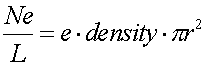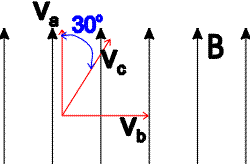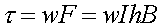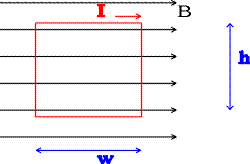Examples for magnetic fields and forces

Example #1

Problem:

A current of 10.0 amps moves through a wire of diameter, d = 3.0 mm. The density of free electrons in the wire is 5.0E27 electrons/m3. What is the average velocity of the electrons in the current?

Solution:

The current in a wire is given by the charge per unit length times the velocity of the charge.The charge per unit length is the charge per volume times the cross-sectional area of the wire.One can then solve for the velocity using the first equation.

1.77E-3 m/s, pretty slow!

Example #2

Problem:

In the figure below, the magnetic field has a strength of 0.75 T. Three charged particles a, b, and c, each with a velocity of 500 m/s and charge of 4.0 mC travel with the directions shown. What is the magnitude of the force on each particle?Solution:Fa = 0, Fb = 1.5E-3 N , Fc = 7.5E-4 N

Example #3

Problem:

In the figure below, what are the net force and torque on the loop?

DATA: I = 3.0 amps, w = 0.5 m, h = 0.7 m, B = 1.5 TSolution:

All the forces cancel out, but there is a force out of the screen from the right segment and a force into the screen on the left segment. These make the loop want to twist, and give it a torque:F = 0, t = 1.575 Nm

Example #4

Problem:

A proton makes a 30 cm circular orbit in a 2.5 T magnetic field. What is the velocity of the proton?

DATA: mass of proton = 1.67E-27 kg, e = 1.602E-19 C.

Solution:

Use the relation:to solve for the velocity.

v = 7.2E7 m/s

Examples       Magnetic fields and forces index        Lecture index